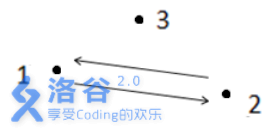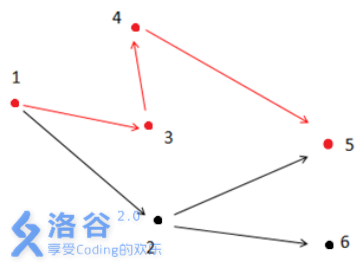# P2296 寻找道路

• 6.8K通过
• 22K提交
• 题目提供者 CCF_NOI
• 评测方式 云端评测
• 标签 图论 搜索 NOIp提高组 2014
• 难度 普及+/提高
• 时空限制 1000ms / 128MB
• 提示：收藏到任务计划后，可在首页查看。

## 题目描述

在有向图 $G$ 中，每条边的长度均为 $1$，现给定起点和终点，请你在图中找一条从起点到终点的路径，该路径满足以下条件：

1. 路径上的所有点的出边所指向的点都直接或间接与终点连通。
2. 在满足条件$1$的情况下使路径最短。

注意：图 $G$ 中可能存在重边和自环，题目保证终点没有出边。

请你输出符合条件的路径的长度。

## 输入输出格式

输入格式：

第一行有两个用一个空格隔开的整数 $n$ 和 $m$，表示图有 $n$ 个点和 $m$ 条边。

接下来的 $m$ 行每行 $2$ 个整数 $x,y$，之间用一个空格隔开，表示有一条边从点 $x$ 指向点$y$。

最后一行有两个用一个空格隔开的整数 $s, t$，表示起点为 $s$，终点为 $t$。

输出格式：

输出只有一行，包含一个整数，表示满足题目描述的最短路径的长度。如果这样的路径不存在，输出$-1$。

## 输入输出样例

输入样例#1： 复制
3 2
1 2
2 1
1 3

输出样例#1： 复制
-1
输入样例#2： 复制
6 6
1 2
1 3
2 6
2 5
4 5
3 4
1 5

输出样例#2： 复制
3

## 说明

解释1：如上图所示，箭头表示有向道路，圆点表示城市。起点$1$与终点$3$不连通，所以满足题目描述的路径不存在，故输出$-1$ 。

解释2：如上图所示，满足条件的路径为$1$- >$3$- >$4$- >$5$。注意点$2$ 不能在答案路径中，因为点$2$连了一条边到点$6$ ，而点$6$ 不与终点$5$ 连通。

【数据范围】

对于$30\%$的数据，$0 < n \le 10$，$0 < m \le 20$;

对于$60\%$的数据，$0 < n \le 100$，$0 < m \le 2000$;

对于$100\%$的数据，$0 < n \le 10000, 0 < m \le 200000,0 < x,y,s,t \le n, x,s \ne t$。

提示
标程仅供做题后或实在无思路时参考。
请自觉、自律地使用该功能并请对自己的学习负责。
如果发现恶意抄袭标程，将按照I类违反进行处理。